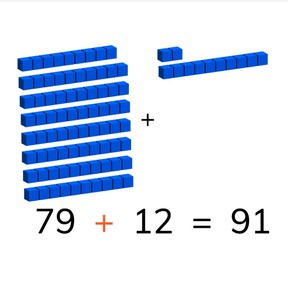No account needed.8,000 schools use Gynzy92,000 teachers use Gynzy1,600,000 students use Gynzy

## General

Students learn to add to 100 crossing tens with addends greater than 10.

2.NBT.B.5
2.OA.A.1

## Relevance

It is important for students to learn to add to 100 crossing tens so that they learn how to calculate total amounts of larger amounts.

## Introduction

First practice on the number line by asking students to drag the balloon with a number to where it belongs. Then practice with to addition problems on the number line to 50 with and without crossing tens.

## Development

Check that students are able to add to 100 by asking the following questions:
- Why is it useful to be able to add to 100?
- What do you look at first when adding to 100?
- How do you regroup tens for example in the problem 58+23?

## Guided practice

Students first practice an addition problem with visual support, then they practice without visual support and finally are given a story problem to solve.

## Closing

Give each student a sheet of paper and tell them they will be making addition problems. They must first write a number between 1 and 50. They walk around the class, find a partner, and then solve the addition problem with their two numbers. Then half of the class writes a number from 10-20, and the other half from 20-80. The students must find a partner from the other half of the class and solve the addition problem with their two numbers.

## Teaching tips

Students who have difficulty adding to 100 can be supported by the use of manipulatives. They can also be helped by writing out the addition problems on the number line.

## Instruction materials

Paper to create number cards.

### The online teaching platform for interactive whiteboards and displays in schools

• Save time building lessons

• Manage the classroom more efficiently

• Increase student engagement